Freight train

The train carries 525 tons of limestone in 29 wagons. Wagons are 15 tonne and 20 tons. How many is 15 ton and how many is 20 ton wagons?

Result

x =  11
y =  18

Solution:

x+y=29
15 x + 20 y = 525

x+y = 29
15x+20y = 525

x = 11
y = 18

Calculated by our linear equations calculator.

Leave us a comment of this math problem and its solution (i.e. if it is still somewhat unclear...):Be the first to comment!To solve this verbal math problem are needed these knowledge from mathematics:

Do you have a system of equations and looking for calculator system of linear equations?

Next similar math problems:

1. Barrel 4Barrel of water weighs 63 kg. After off 75% water, the weight of the barrel with water is 21 kg. How many kg weigh empty barrel and how many kgs water in it?
2. Tram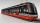In the three-part tram went 206 passengers in front of others, 226 after others and in the middle half of all. How many passengers went in tram total?
3. TheatroTheatrical performance was attended by 480 spectators. Women were in the audience 40 more than men and children 60 less than half of adult spectators. How many men, women and children attended a theater performance?
4. Three daysDuring the three days sold in stationery 1490 workbooks. The first day sold about workbooks more than third day. The second day 190 workbooks sold less than third day. How many workbooks sold during each day?
5. Football league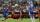In the 2nd football league plays each of the 16 participants with each opponent twice. For every victory gets 3 points, 1 point for a draw, a defeat none. After the competition was team with 54 points and 12 defeats at 9th place. How many times have won?
6. Waiting roomIn the waiting room are people and flies. Together they have 15 heads and 50 legs (fly has 6 legs). How many people and flies are in the waiting room?
7. Buttons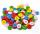Shirt has 6 buttons, blouse has 4 buttons. All buttons are 176. Shirts and blouses are together 36. How many are the shirts and blouses?
8. Three friendsThe three friends spent 600 KC in a teahouse. Thomas paid twice as much as Paul. Paul a half less than Zdeněk. How many each paid?
9. Donuts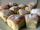In the dining room at one table sit Thomas, Filip and Martin. Together they eat 36 donuts. How many donuts eaten everyone, when Filip ate twice as much as Thomas and Martin even half over Filip?
10. MushroomsEva and Jane collected 114 mushrooms together. Eve found twice as much as Jane. How many mushrooms found each of them?
11. Pages of book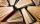Lenka calculated that if every day to read 16 pages of the book, read a book a day earlier than had read only 14 pages a day. How many pages has this book?
12. Three brothersThe three brothers have a total of 42 years. Jan is five years younger than Peter and Peter is 2 years younger than Michael. How many years has each of them?
13. EquationsSolve following system of equations: 6(x+7)+4(y-5)=12 2(x+y)-3(-2x+4y)=-44
14. Two numbersWe have two numbers. Their sum is 140. One-fifth of the first number is equal to half the second number. Determine those unknown numbers.
15. Equations - simpleSolve system of linear equations: x-2y=6 3x+2y=4
16. Linear systemSolve this linear system (two linear equations with two unknowns): x+y =36 19x+22y=720
17. Magic numberThe number 135 split to two addends so that one addend was 30 greater than 2/5 the addend.